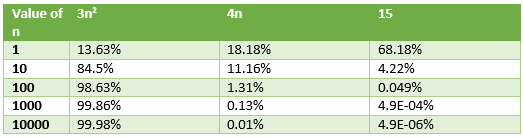© 2021 - First Crazy Developer (Abhishek Kumar)

###Rules to find the Big O Notation

Today we will discuss the finding Big O Notation value. There are certain rules which help us to find that value. We will discuss the rules with example. Look at the following rules:

• Keep the fastest growing term and discard the lower terms and constants value

Example: Suppose we have following function

f(n)= 3n2  + 4n + 15 here f(n) is O(g(n))

if n=10 =>> f(n)=300 +40+15

Now we calculate the growing % term

For 3n2  =>> (300/300+40+15)*100 = 84.5 %

For 4n =>> (40/300+40+15)*100 = 11.26%

For 15 =>> (15/300+40+15)*100= 4.22%

Look at the following table:If we analyse the above table we can see that as per value growth of n the 3n2 value is dominate the function value and 4n is the smaller term and 15 is constant term become insignificant.

This is happening due to n2 grows more rapidly than n and constant doesn’t grow at all.

After all the above discussion we found:

Big O analysis => Value of function as n becomes large. When n becomes large then Fastest growing term becomes dominant. Lower and Constant terms becomes insignificant.

• Ignore Coefficients

Example:

If we have following function=> f(n)=C*g(n)=>> f(n) is O(g(n))

In the above example C is constants value so we can say that f(n) is order of g. Like

f(n)=5n2 then we can say  f(n) = O(n2 )

f(n) = 10n then we can say f(n) = O(n)

f(n)= 3n2  + 4n + 15

So after first rule the function become

f(n)= 3n2  >> we ignored the lower and constants terms

After second rule the function become

f(n) = O(n2)

Look at the following final output in a line:

f(n)= 3n2  + 4n + 15 => f(n)= 3n2  =>> f(n) = O(n2)

•  If f(n) is constants, then we can say that f(n) is O(1)

f(n) =C => f(n) is O(1)

Look at the following example:

f(n)=3 then then function is order of 1 =>  f(n) = O(1)

f(n)=500 then then function is order of 1 =>  f(n) = O(1)

f(n)=100000 then then function is order of 1 =>  f(n) = O(1)

•  When we compute the value of O notation then base of logarithm is not important

f(n) =8log2n, the f(n) => O(logn)

Due to base of logarithm denote the constants factor and in O natation we ignore the constants factors.

After all the above rules we do some practice with the help of above rules:

a.)    f(n)=45 become O(1)

b.)    f(n)= 7n3+27log2n+2n  become O(n3)

c.)     f(n)=8log2n+7n+56 become O(n)

d.)    f(n) = 70log2n+16n+34n2 become O(n2)

e.)    f(n)= 2logn+nlog10n become O (n log n)

f.)     f(n)= 3n+5n2+7n3+2n become O(2n)

g.)    f(n)= 7n+ 2n2+ 5n + n! become O(n!) here n factorial is larger value than otherHome Page 26 August 2017